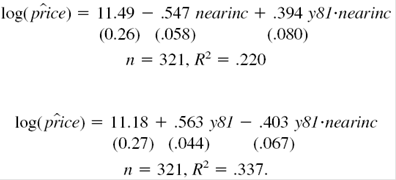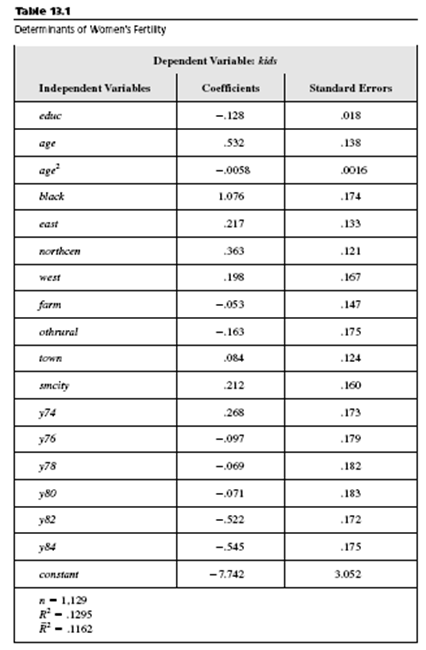### Create an Account

Home / Questions / In Example 13.1 assume that the average of all factors other than educ have remained const...

# In Example 13.1 assume that the average of all factors other than educ have remained constant over time and that the average level of education

In Example 13.1, assume that the average of all factors other than educ have remained constant over time and that the average level of education is 12.2 for the 1972sample and 13.3 in the 1984 sample. Using the estimates in Table 13.1, find the estimated change in average fertility between 1972 and 1984. (Be sure to account for the intercept change and the change in average education.) Using the data in KIELMC.RAW, the following equations were estimated using the years 1978 and 1981:Compare the estimates on the interaction term y81_nearinc with those from equation

(13.9). Why are the estimates so different?May 02 2020 View more View LessSubscribe To Get Solution